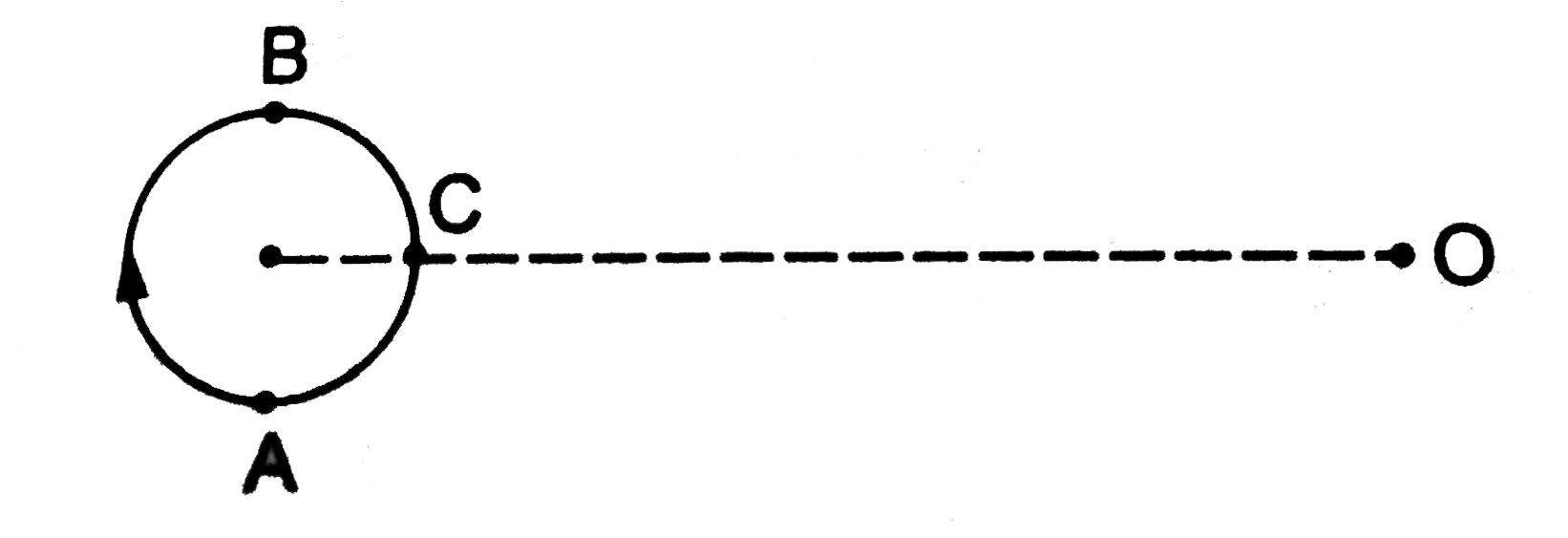# A small source of sound moves on a circle as shown in figure and an observer is sitting at O. Let v_1,v_2,v_3 be the frequencies heard when the sour

41 views
in Physics
A small source of sound moves on a circle as shown in figure and an observer is sitting at O. Let v_1,v_2,v_3 be the frequencies heard when the source is at A,B and C respectively..
A. upsilon_(1) gt upsilon_(2) gt upsilon_(3)
B. upsilon_(1) = upsilon_(2) gt upsilon_(3)
C. upsilon_(2) gt upsilon_(3) gt upsilon_(1)
D. upsilon_(1) gt upsilon_(3) gt upsilon_(2)

by (85.3k points)